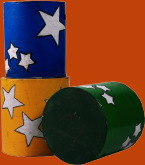# How To Calculate The Volume Of A CylinderCalculating the volume of a cylinder is even easier than calculating its area. All you have to do is recognize that a cylinder is no more than just a bunch of circles stacked to a certain height, just like a cube is just a bunch of squares stacked up one on top of the other, and every circle in the stack is identical. The area of each circle is obtained by multiplying the radius of the circle by itself and then by the value of p. The length of the cylinder is just the length of the cylinder. The volume of the cylinder can then be calculated by the general formula: V = pr2l.

As an example of how to use this formula, suppose you have a large water storage tank by your garden that you need to fill with water. You also promised to take the kids to a baseball game in exactly four hours. Your garden hose delivers 25 liters of water every minute, and you want to know how long it will take to fill the tank this way The tank is three meters long and 1.5 meters wide, which means the radius is 0.75 meters. (use p = 3.1416 for a more accurate estimate). The volume is therefore; V = pr2, = 3.1416 X 3 X 0.75 X 0.75, = 5.8905 cubic meters.

Each cubic meter contains 1000 liters, so the tank holds 5890.5 liters. At 25 liters per minute it will take 235.62 minutes to fill the tank from your garden hose, or 3 hours and 56 minutes. Turn the hose on right now, and don't forget to turn it off when you take the kids to the baseball game.

#### About the Author

Richard M J Renneboog, MSRichard M. J. Renneboog is an independent private technical consultant and writer in both chemical and computer applications. Endeavors have included preparation of scripts for instructional and promotional video, corporate website design, curriculum development for training in advanced composites technology, and development.

### Our Sponsors: